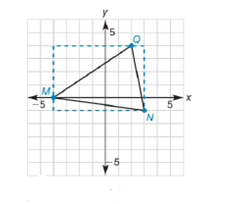Chapter 10.1, Problem 33E### Elementary Geometry for College St...

6th Edition
Daniel C. Alexander + 1 other
ISBN: 9781285195698

#### Solutions

Chapter
Section### Elementary Geometry for College St...

6th Edition
Daniel C. Alexander + 1 other
ISBN: 9781285195698
Textbook Problem
1 views

# The triangle that has vertices at M - 4 ,   0 ,   N 3 ,   - 1 ,     a n d   Q ( 2 ,   4 ) has been boxed in, as shown. Find the area of ∆ M N Q .Exercises 33, 34.

To determine

To find:

The area of MNQ and the vertices are M-4, 0, N3, -1, and Q(2, 4).

Explanation

Given vertices are,

M-4, 0, N3, -1, and Q(2, 4)

Given figure is,

Sketch the points and draw the triangle in the graph as shown in figure,

Base of the triangle can be determined by subtracting x-coordinates of MN

Base MN=3--4=7

Height of the triangle can be determined by subtracting

### Still sussing out bartleby?

Check out a sample textbook solution.

See a sample solution

#### The Solution to Your Study Problems

Bartleby provides explanations to thousands of textbook problems written by our experts, many with advanced degrees!

Get Started

#### 1. Convert the constraint inequalities to equations containing slack variables.

Mathematical Applications for the Management, Life, and Social Sciences

#### What is the future value of an annuity? Give an example.

Finite Mathematics for the Managerial, Life, and Social Sciences

#### Let and g(x) = x + 3. Then (g ∘ h)(x) = ______.

Study Guide for Stewart's Single Variable Calculus: Early Transcendentals, 8th